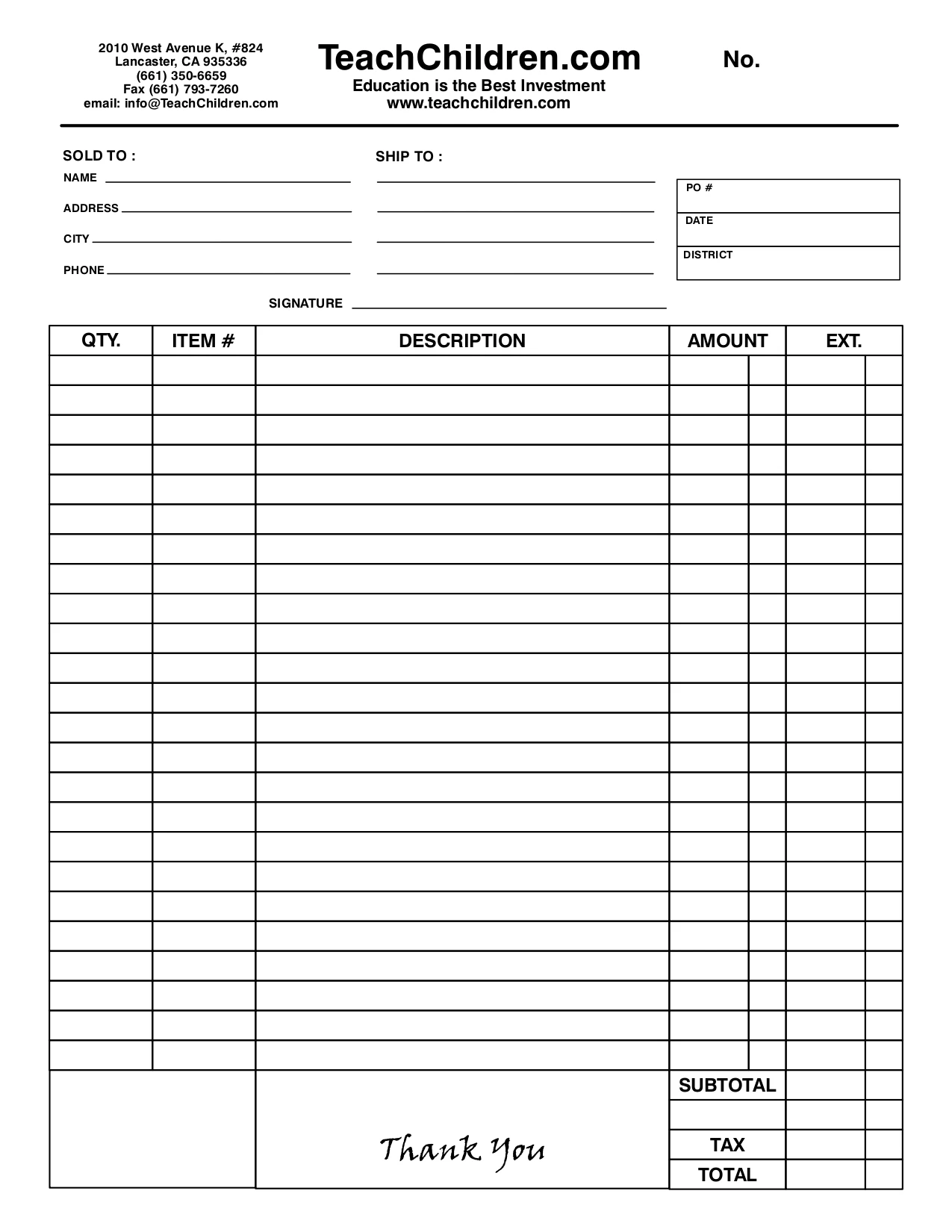×# Solve-the-Problem Mini Books: Fractions & Decimals

(No reviews yet)
SKU:
SC36590
UPC:
9.78E+12
\$14.99

## Description

Research shows that an effective way to teach math concepts is through problem solving. The mini-stories in this book model how students can solve math problems by working together to ask questions, share ideas and strategies, apply prior knowledge to find solutions, and more. Each story comes with follow-up practice problems. Skills include comparing fractions, finding equivalent fractions, using decimal equivalents to compare fractions, adding and subtracting fractions, and more.

Key Features :

i : Math story problems model various strategies for solving the problems.
ii : Focuses on problem types, strategies, and tips for engaging students in making sense of problems
iii : Meets core math standards
View AllClose
×